# The HPMIXED Procedure

### MODEL Statement

• MODEL dependent = <fixed-effects> </ options>;

The MODEL statement names a single dependent variable and the fixed effects, which determine thematrix of the mixed model. The specification of effects is the same as in the GLM procedure; however, unlike PROC GLM, you do not specify random effects in the MODEL statement. The MODEL statement is required.

An intercept is included in the fixed-effects model by default. If no fixed effects are specified, only this intercept term is fit. The intercept can be removed by using the NOINT option.

You can specify the following options in the MODEL statement after a slash (/).

ALPHA=number

requests that a t-type confidence interval be constructed for each of the fixed-effects parameters with confidence level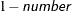. The value of number must be between 0 and 1; the default is 0.05.

CL

requests that t-type confidence limits be constructed for each of the fixed-effects parameter estimates. The confidence level is 0.95 by default; this can be changed with the ALPHA= option.

DDF=value-list

enables you to specify your own denominator degrees of freedom for the fixed effects. The value-list specification is a list of numbers or missing values (.) separated by commas. The degrees of freedom should be listed in the order in which the effects appear in the "Type III Tests of Fixed Effects" table. If you want to retain the default degrees of freedom for a particular effect, use a missing value for its location in the list. For example, the following statement assigns 3 denominator degrees of freedom to A and 4.7 to A*B, while those for B remain the same:

model Y = A B A*B / ddf=3,.,4.7;

DDFM=RESIDUAL | NONE

specifies the method for computing the denominator degrees of freedom for the tests of fixed effects resulting from the MODEL, CONTRAST , ESTIMATE , LSMEANS , and TEST statements.

The DDFM=RESIDUAL option performs all tests by using the residual degrees of freedom,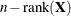, where n is the number of observations used. It is the default degrees of freedom method.

DDFM=NONE specifies that no denominator degrees of freedom be applied. PROC HPMIXED then essentially assumes that infinite degrees of freedom are available in the calculation of p-values. The p-values for t tests are then identical to p-values derived from the standard normal distribution. In the case of F tests, the p-values equal those of chi-square tests determined as follows: if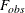is the observed value of the F test with l numerator degrees of freedom, then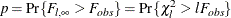NOINT

requests that no intercept be included in the model. An intercept is included by default.

SOLUTION | S

requests that a solution for the fixed-effects parameters be produced. Using notation from the section Model Assumptions, the fixed-effects parameter estimates are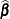and their approximate standard errors are the square roots of the diagonal elements of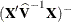.

Along with the estimates and their approximate standard errors, a t statistic is computed as the estimate divided by its standard error. The degree of freedom for this t statistic matches the one appearing in the "Type III Tests of Fixed Effects" table under the effect containing the parameter. The "Pr > |t|" column contains the two-tailed p-value corresponding to the t statistic and associated degrees of freedom.

ZETA=number

tunes the sensitivity in forming Type III functions. Any element in the estimable function basis with an absolute value less than number is set to 0. The default is 1E–8.